# Uniswap Labs Blog

The Dominance of Uniswap v3 Liquidity

May 05, 2022

Gordon Liao and Dan Robinson[^1]

[^1]: We thank Austin Adams for assisting with the research. We are grateful for comments from Hayden Adams and Matteo Leibowitz.

New research published today shows that Uniswap Protocol v3 has deeper liquidity in ETH/USD, ETH/BTC and other ETH pairs than leading centralized exchanges. This research demonstrates that AMM market structure – which is largely crypto-native today – can surpass order-book exchanges and transform traditional financial market structure to be more liquid, stable, and secure.

The complete research report and our open-sourced methodology are below (and in pdf form here), and we are making our code and data freely available. In summary:

• For ETH/USD, Uniswap has ~2x more liquidity than both Binance and Coinbase.
• For ETH/BTC, Uniswap has ~3x more liquidity than Binance and ~4.5x more liquidity than Coinbase.
• For ETH/mid-cap pairs Uniswap has on average ~3x more liquidity than major centralized exchanges.
Market depth comparison for ETH/USD stables and ETH/BTC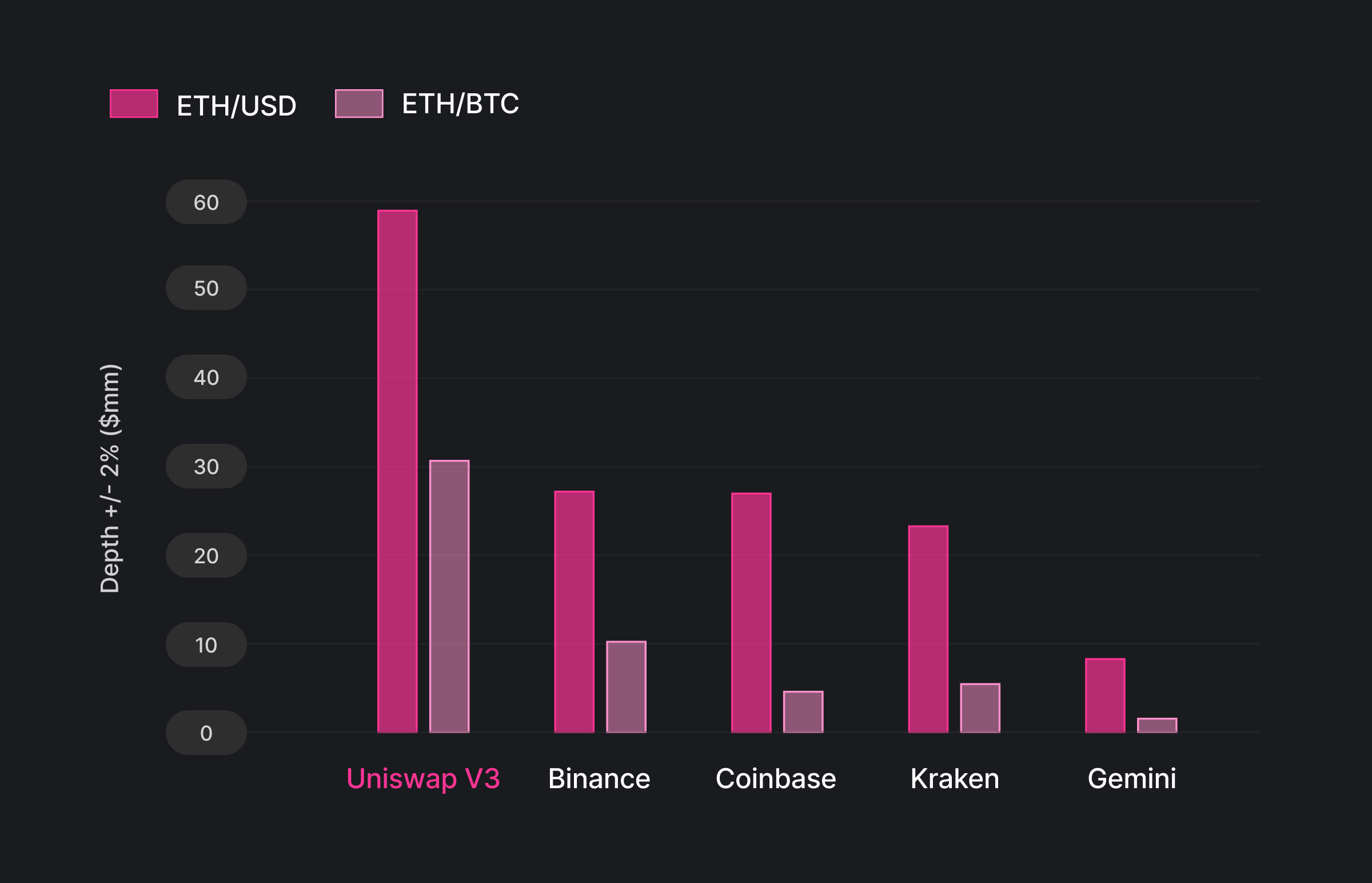The figure shows the daily average +/- 2% spot market depth in $millions for the sample period from June 2021 to March 2022 for ETH/USD and February 2022 to March 2022 for ETH/BTC. The market depth for ETH/USD is aggregated across ETH/USD (fiat), ETH/USDC, ETH/USDT, and ETH/DAI. Data on centralized exchanges is provided by Kaiko. The comparison does not include some exchanges, including FTX and Bybit, due to a lack of data provided by the exchange. The research also finds that many stablecoin pairs have much more liquidity on Uniswap v3 than centralized exchanges. For USDC/USDT, Uniswap v3 has about ~5.5x more liquidity than Binance. Uniswap v3 also has higher market depth across all price levels, which means it’s even more advantageous for users to execute larger trades on Uniswap v3 relative to centralized exchanges. For an ETH/dollar trade size of$5mm, for example, the savings would be around $24,000 given the expected price impact difference. (For$5mm notional, the average price impact is roughly 0.5% on Uniswap v3 and 1% on Coinbase. The fee is about 2 bps lower on Coinbase on average.)

Traditional market structure is dominated by a few market makers. But easy liquidity creation (AMMs) dramatically lowers the barrier to create and participate in markets. This unlocks new and existing forms of value for communities and individuals.

We look forward to sharing more research with you!

# The Dominance of Uniswap v3 Liquidity

Automated Market Makers (AMMs) have emerged as an important form of liquidity provision for digital assets. The typical explanation for why some market participants have opted to use AMMs over centralized exchanges is that AMMs enable composability with other decentralized financial (DeFi) protocols and provide trading without asset custody by intermediaries. One less examined, but perhaps more important, reason for why AMMs might dominate in the future of trading for both digital and non-digital-native assets is that AMMs can deliver higher liquidity than centralized exchanges. This is because the decentralized AMM design reduces technical barriers to market making and opens the floodgates to let in an ocean of previously unused capital.

We compare the liquidity on Uniswap v3 (white paper), the most popular AMM, with that of centralized exchanges, across a set of large-cap digital asset pairs. We find that Uniswap v3 consistently has substantially higher liquidity, as measured by market depth, compared to the largest centralized exchanges in spot markets.

Figure 1 illustrates our main finding. Compared to several large centralized exchanges, Uniswap v3 has around 2x greater market depth on average for spot ETH-dollar pairs (using the most liquid dollar or stablecoin on each platform for comparison). Uniswap v3 compares even more favorably by aggregating risk across all ETH-dollar pairs, as shown in Appendix Figure A1.

Figure 1. Market depth comparison for ETH/USD stables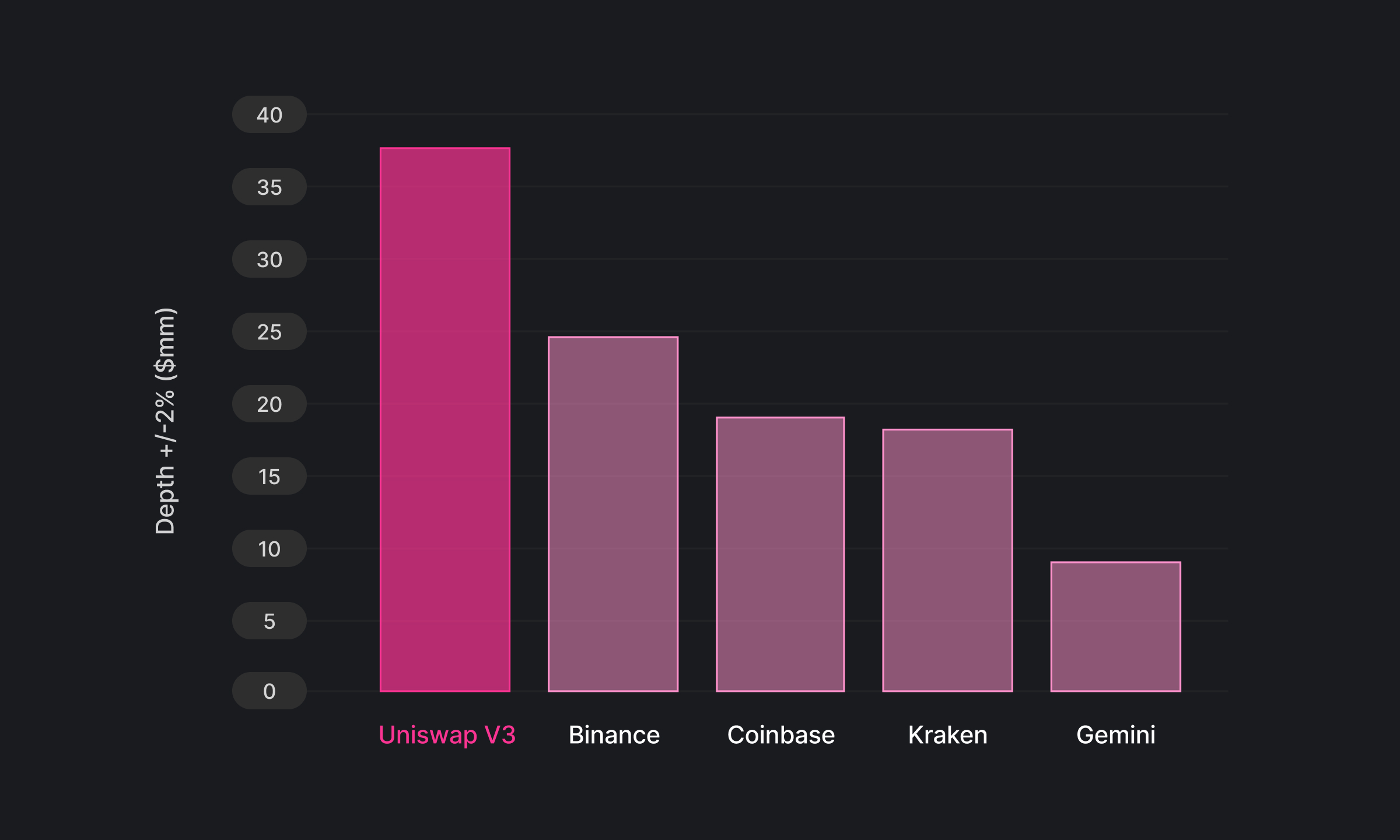The plot shows the daily average +/- 2% spot market depth in $millions for the sample period from June 1, 2021 to March 1st, 2022. The comparison uses the most liquid ETH/USD pair for each of these markets (ETH/USDC on Uniswap v3 Layer 1, ETH/USDT on Binance, ETH/USD on Coinbase, and ETH/USD on Gemini). The market depth data for centralized exchanges is provided by Kaiko. The comparison does not include some exchanges, including FTX and Bybit, due to a lack of data provided by the exchange. Beyond ETH/USD pairs, Uniswap v3 also has greater market depth for ETH-based mid-cap token pairs as well as stablecoin-to-stablecoin pairs. ## Measuring liquidity via market depth Market depth is a common measure of liquidity for assets traded on exchanges. At its core, market depth indicates how much of one asset can be traded for another asset at a given price level. High market depth is needed to support high transaction volumes with reliable execution. Market depth in traditional limit order books can be calculated by adding up the amount of limit order at each price level. For instance, Coinbase provides market depth charts for its pro-account users as shown in Figure 2. Figure 2. Market depth chart on Coinbase Pro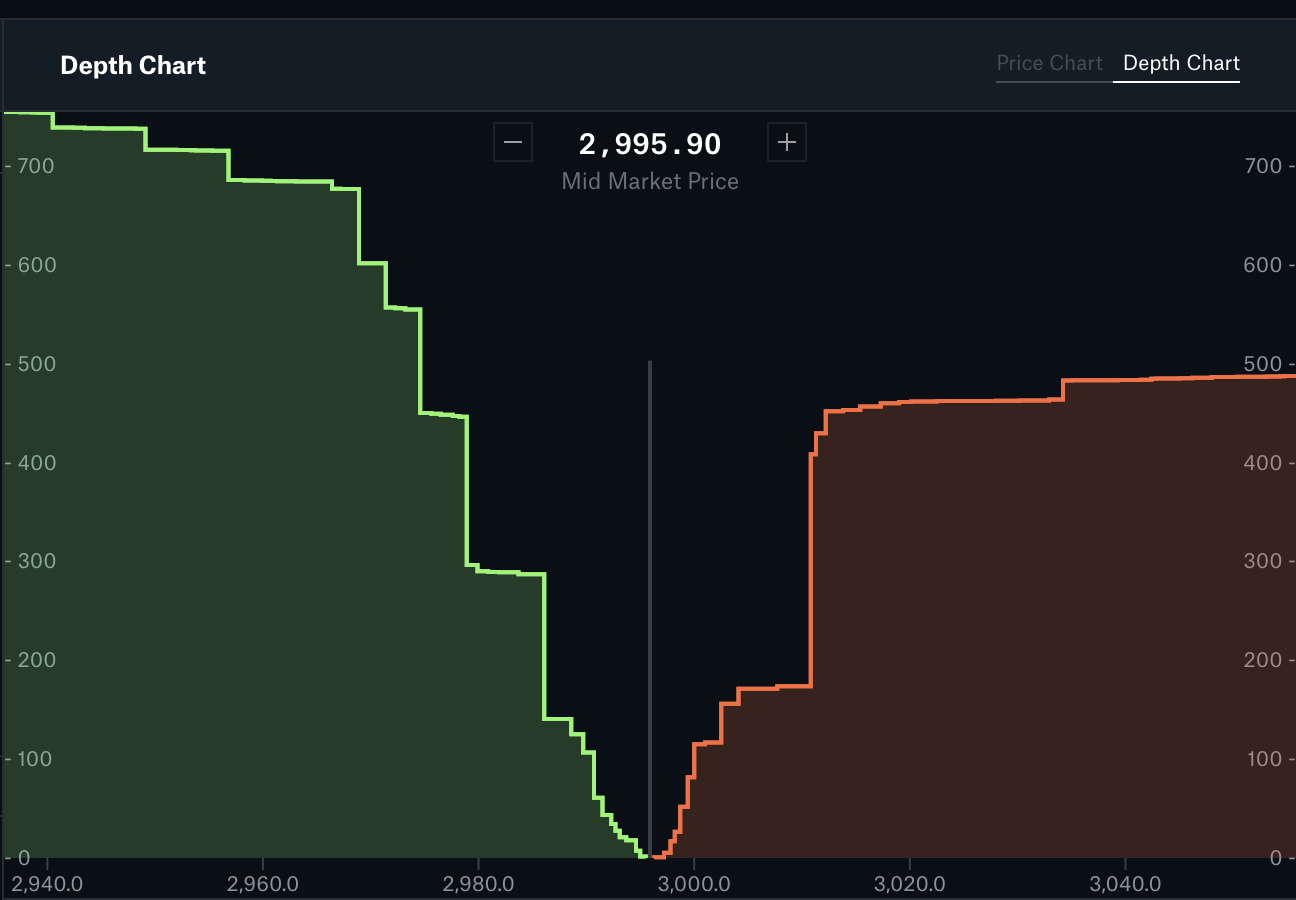In AMMs, there are no limit order books. Instead, liquidity providers (LPs) deposit a pair of assets into a common pool that can be traded against by liquidity demanders (traders). In exchange for their capital and the risk they take on due to potential adverse selection, liquidity providers earn a fee on every trade. LPs can post assets into a liquidity pool either in a customizable range of prices (Uniswap v3) or in the full price range (Uniswap v2). The distribution of liquidity over a price range provides the signature necessary to derive an equivalent market depth similar to that of limit order books. We provide a methodology for calculating market depth on Uniswap v3 (see appendix below) and implement the calculation across pools and fee tiers. We’ve made both our code and data freely available.[^2] [^2]: We provide daily market depth calculated on the top 1,000 liquidity pools by historical volume and the code to generate this data. ## Comparison of Uniswap v3 versus centralized exchanges Overall, Uniswap v3 has had significantly higher market depth throughout the past several months than centralized exchanges in spot trading. This higher liquidity is observed on both large-cap pairs such as ETH/USDC (relative to equivalent pairs on centralized exchanges) and mid- to small-cap pairs. Figure 3 below shows the time series of ETH-dollar pairs across Uniswap v3, Binance, and Coinbase. Figure 3. Market depth comparison for Uniswap v3 (ETH/USDC), Binance (ETH/USDT), and Coinbase (ETH/USD)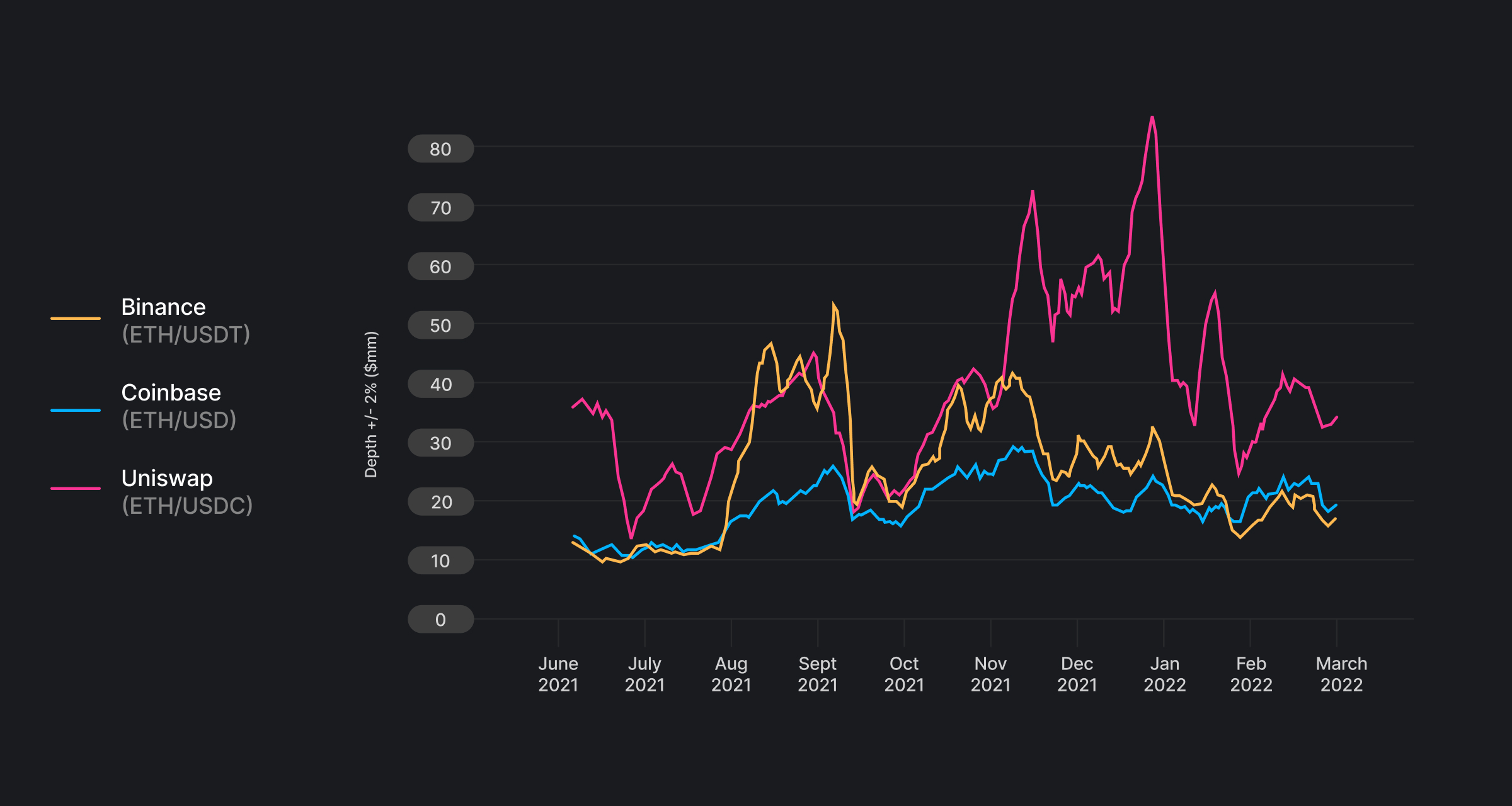The time series is of 7-day rolling mean of +/- 2% spot market depth in$millions. Market depth on Uniswap v3 is from Layer 1 only. The market depth data for centralized exchanges is provided by Kaiko.

Similar to ETH/dollar pairs, Uniswap v3 also has higher average market depth on mid-cap and cross-chain pairs (Table 1) as well as stablecoin-to-stablecoin pairs (Appendix Figure A2).

Table 1. Liquidity comparison for ETH-pairs (in units of ETH for +/-2% depth)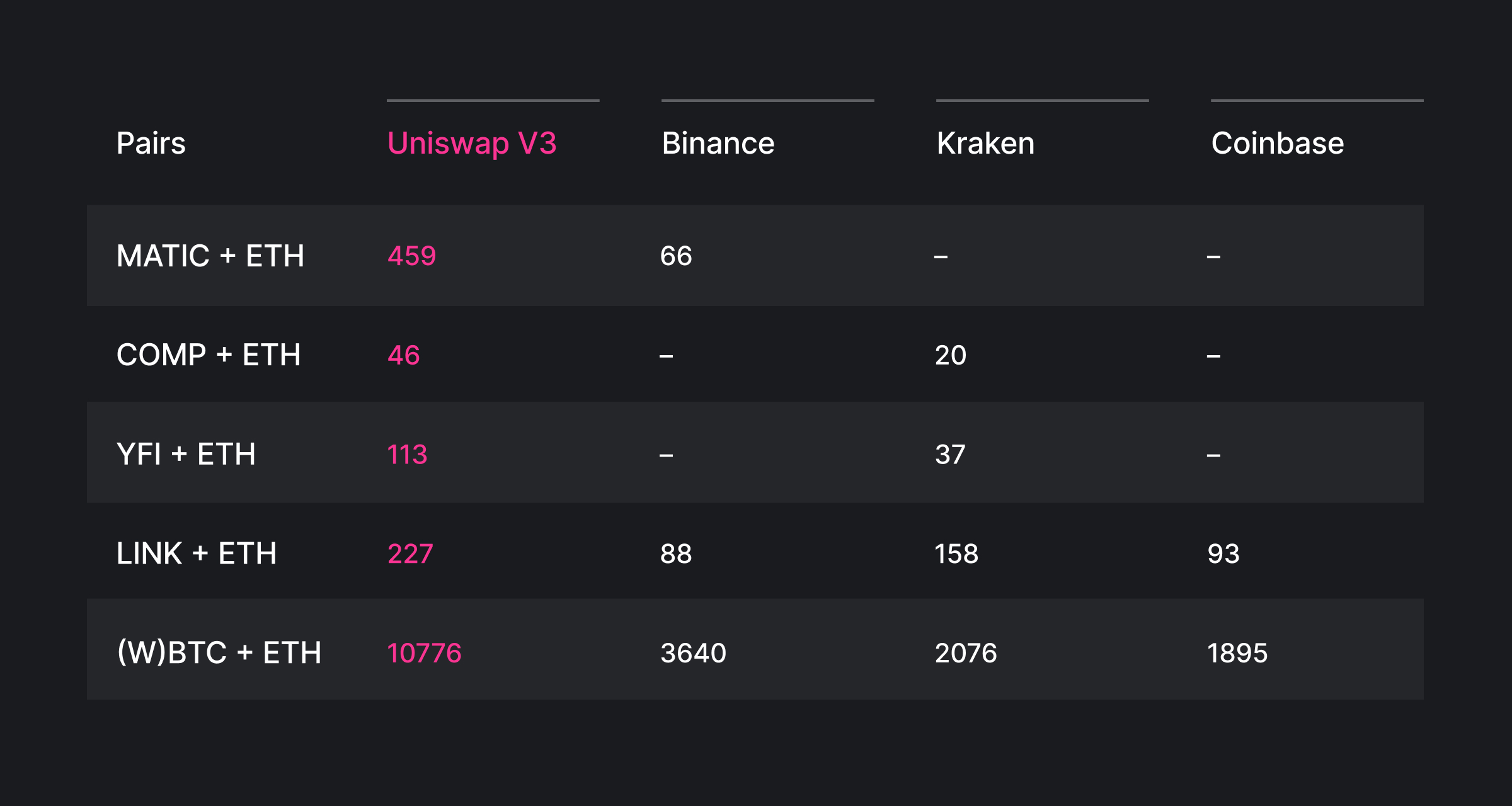The table shows +/- 2% average spot market depth in units of ETH in the sample period 02/28/22-03/28/22. Market depth on Uniswap v3 is from Layer 1 only. The market depth data for centralized exchanges is provided by Kaiko.

Additionally, higher market depth on Uniswap v3 is observed across all price levels, as seen in Figure 4. The liquidity advantage for Uniswap v3 grows with the expected price impact. This means that for larger trades, it is even more advantageous to execute on Uniswap v3 relative to centralized exchanges.

Figure 4. Market depth comparison for Uniswap v3 ETH/USDC vs Coinbase ETH/USD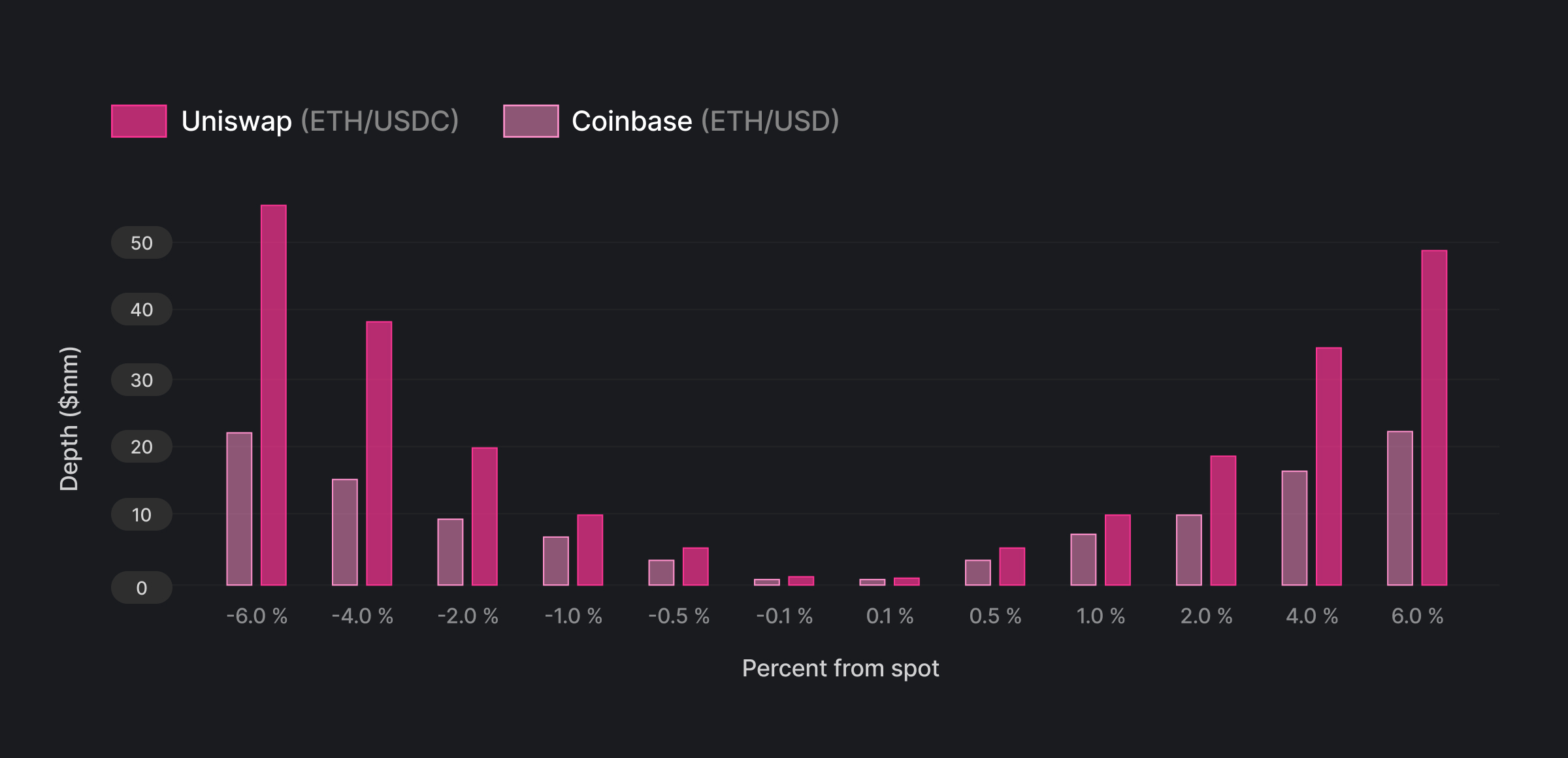The plot shows the average spot market depth in $millions for the sample period from June 1, 2021 to March 1st, 2022 across price levels. Market depth on Uniswap v3 is from Layer 1 only. The market depth data for Coinbase is provided by Kaiko The above analysis does not take into account transaction costs, including gas cost on Ethereum, fees on centralized or decentralized exchanges, or unexpected pre-trade slippage. These are potential topics for future research. Broadly speaking, these additional transaction fees are comparable for Uniswap v3 and centralized exchanges and are small relative to costs associated with market depths for large trade sizes.[^3] [^3]: Fees Uniswap v3 has several fee tiers ranging from 1 bp that are popular for stablecoin-only pairs to 100 bps for the most volatile long-tail assets. Centralized exchanges charge transaction fees as well. For instance, Coinbase Pro has fees that range from 5 bps to 60 bps depending on the monthly transaction volume. Additionally, centralized limit order books have bid/ask spreads that vary based on the market and time. Gas cost On-chain transactions through AMMs have gas costs that depend on network congestion. The median cost of a swap transaction on Uniswap v3 has been$31 per trade since its inception.

The cost savings from executing on a more liquid market is direct. For a trade on the ETH/dollar pair that results in a 5 bps average price impact in Coinbase Pro, the equivalent trade on Uniswap v3 would roughly generate a 3.8 bps price impact on average, saving the trader 1.3 bps of the notional (~0.6 bps net of gas cost).[^4] The savings are even greater for larger trade sizes. For a trade size of $5mm, the savings would be around 0.5% of the notional given the expected price impact difference.[^5] [^4]: A ETH/dollar trade executed on Coinbase Pro that results in an average price impact of 0.05% (corresponding to a notional size of$650,000 at 0.1% market depth assuming linear scaling of price impact with notional) would only have an average price impact of 0.0375% on Uniswap v3. This is equivalent to a dollar saving of around $81 dollars on the price impact and around$36 of saving net of gas and fees. The fees are similar on v3 and Coinbase Pro and the average gas cost is $45. For Uniswap v3, the weighted average of fee tiers is around 20 bps. For Coinbase Pro, the fee is 20 bps for the 650k - 1m volume tier. [^5]: For$5mm notional, the average price impact is roughly 0.5% on Uniswap v3 and 1% on Coinbase. The fee is about 2 bps lower on Coinbase.

## Deeper on-chain markets

The decentralized nature of AMMs and more passive liquidity provision opened up market making activities to a broader set of market participants. In traditional centralized exchanges, market making is dominated by high-frequency traders that compete in both the quality of the market making algorithm as well as execution speed, leading to an unnecessary arms race in trading technology and suboptimal market structure.[^6] Batched auctions instead of continuous limit order books could resolve some of the issues associated with the competition for speed, but market making would be still reserved for high frequency traders.

[^6]: Budish, E., Cramton, P., & Shim, J. (2015). The high-frequency trading arms race: Frequent batch auctions as a market design response. The Quarterly Journal of Economics, 130(4), 1547-1621.

AMMs unlock a much larger set of more passive capital that was previously sitting on the sidelines. This is because general institutional and retail users have risk-and-return profiles that differ from those of specialized market makers on centralized exchanges. As a result, AMMs are able to attract more diverse capital sources to participate as market makers and hence deepen liquidity. In earlier versions of AMMs, e.g. Uniswap v2, passive liquidity providers can enter a market making position without any additional intervention. Uniswap v3 introduced concentrated liquidity that requires some rebalancing. Even with rebalancing needs, the vast majority of capital deployed for liquidity provision on v3 are relatively passive. Table 2 shows that around two-thirds of liquidity positions are held greater than one day. Overall, the Uniswap protocol has attracted around 130,000 unique liquidity providers.[^7]

Table 2. Duration of Uniswap v3 LP Positions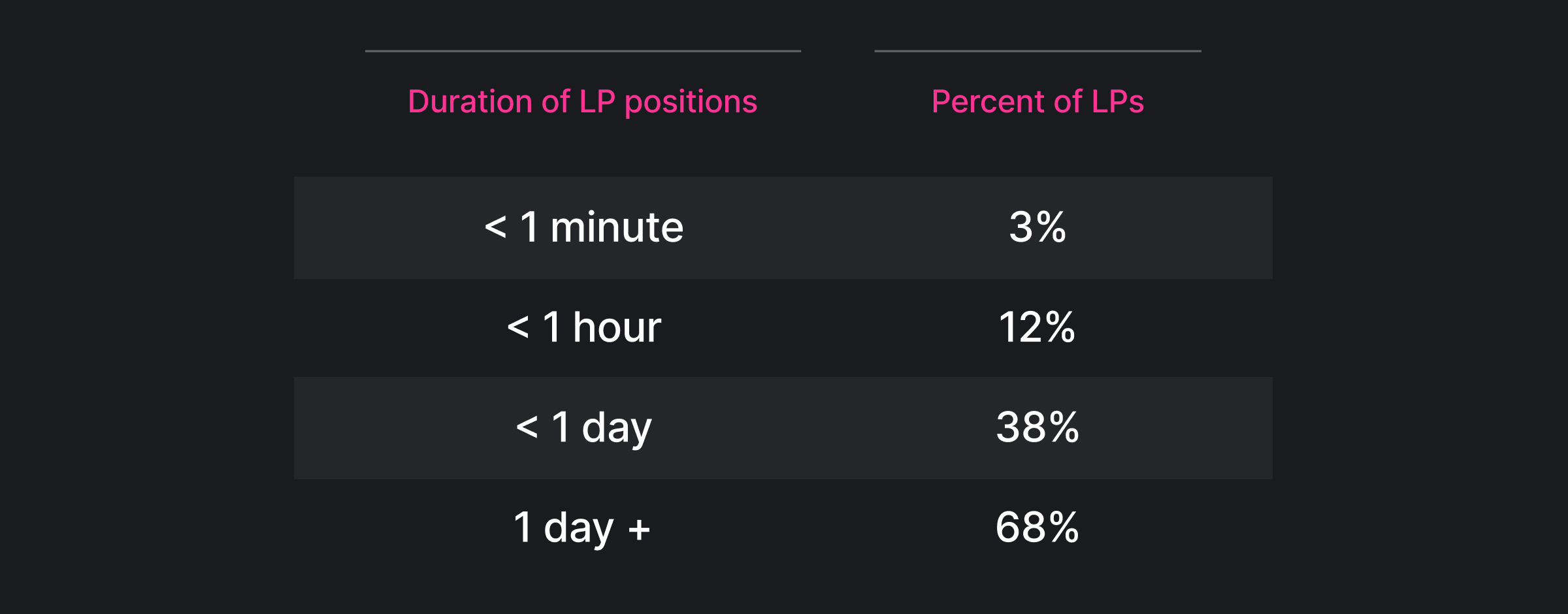The duration of LP positions is the time period between the initial mint and first burn of a LP position.

## Beyond digital assets

Decentralized AMMs is an innovation in market structure with impact far beyond digital assets. The lack of liquidity in thinly traded assets has been a recognized challenge to central limit order exchanges.[^8] This emphasis for innovation in market structure exists because liquidity challenges can be detrimental to both investors and issuers. For investors, low liquidity not only increases transaction costs but also deters willingness to invest in assets that might have high expected returns.[^9] For issuers, poor market liquidity can raise the cost of capital[^10] and increase the chance of default.[^11]

[^8]: “Commission Statement on Market Structure Innovation for Thinly Traded Securities”. Security and Exchange Commission. 17 CFR Part 240 (2019). [^9]: O'Hara, M. (2003). Presidential address: Liquidity and price discovery. The Journal of Finance, 58(4), 1335-1354. [^10]: Butler, A. W., Grullon, G., & Weston, J. P. (2005). Stock market liquidity and the cost of issuing equity. Journal of Financial and Quantitative Analysis, 40(2), 331-348. [^11]: Brogaard, J., Li, D., & Xia, Y. (2017). Stock liquidity and default risk. Journal of Financial Economics, 124(3), 486-502.

In a future with more tokenized assets, the Uniswap Protocol has the potential to not only supplement but also directly compete with traditional exchanges in trading a variety of assets, facilitating both deeper and more stable liquidity. This increase in liquidity can improve the price discovery process for thinly traded assets and ultimately foster capital formation and economic growth.

## Conclusion

Uniswap v3, deployed less than a year ago, has gained dominance over traditional forms of central limit order books in providing a trading venue for deep liquidity in digital assets. As more passive and diverse capital sources are unlocked through decentralized AMMs, market liquidity and efficiency will likely continue to deepen beyond traditional limit order books.

We will highlight other advantages over traditional forms of exchange and price discovery in future studies.

## Appendix A - Additional Figures

Figure A1. Market depth comparison for ETH-dollar pairs (aggregating across pairs)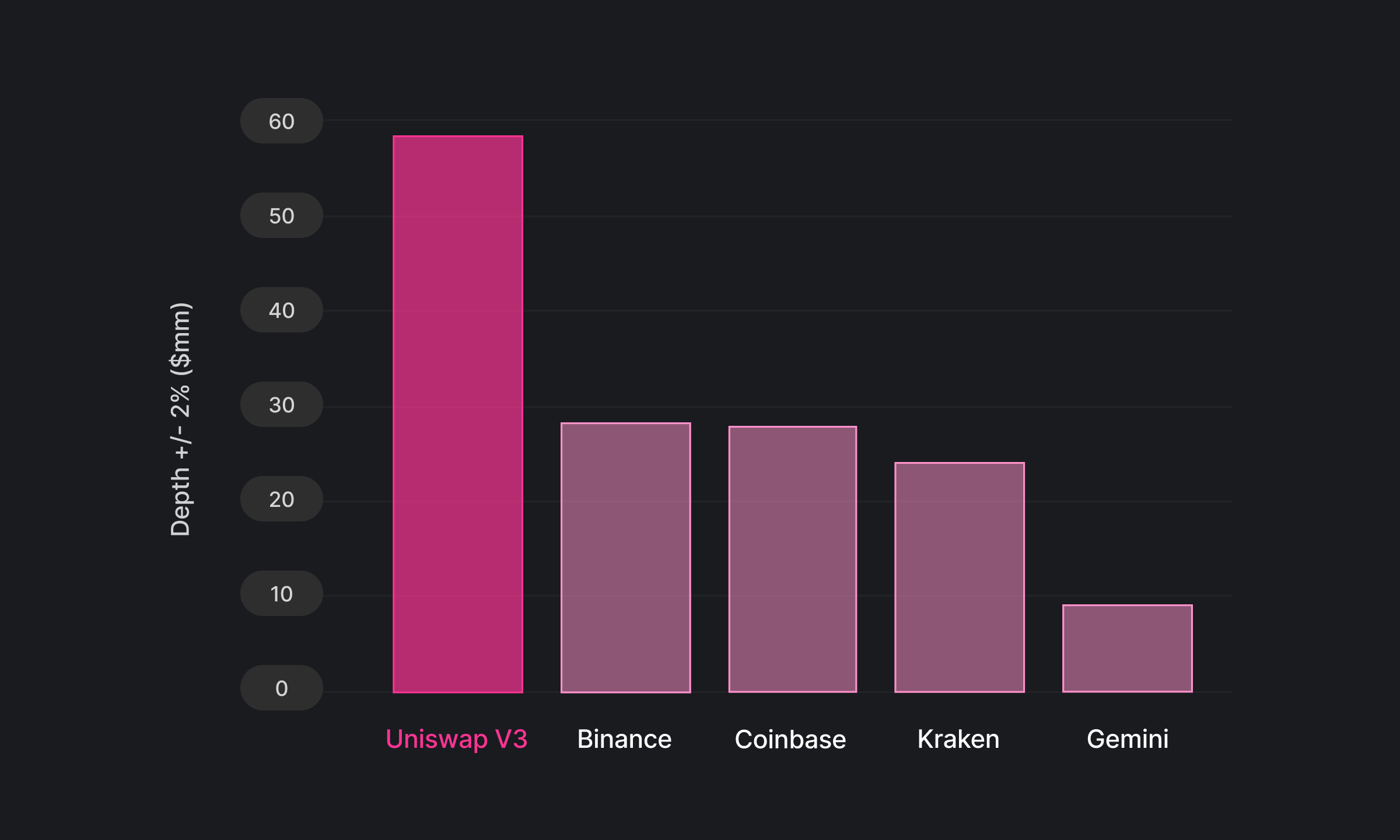This figure compares the market depth for ETH/USD pairs aggregating across ETH/USD, ETH/USDC, ETH/USDT, and ETH/DAI. Data on centralized exchanges is provided by Kaiko. Sample period is from June 2021 to March 2022.

Figure A2. Market depth comparison for USDC/USDT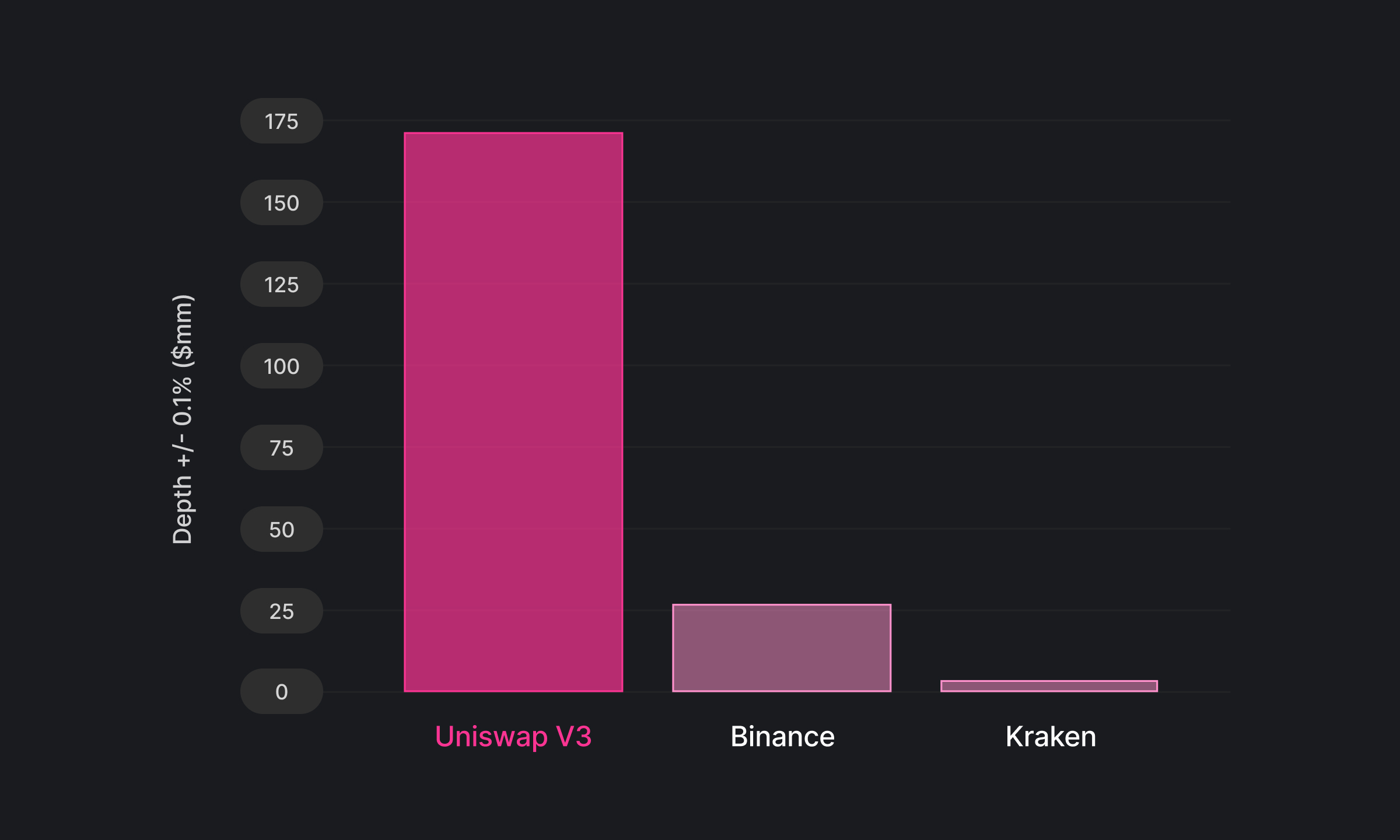This figure compares the market depth for the USDC/USDT stablecoin pair on Uniswap v3, Binance, and Kraken. Data on centralized exchanges is provided by Kaiko. Sample period is from November 2021 to March 2022.

Figure A3. Market depth comparison for (W)BTC/ETH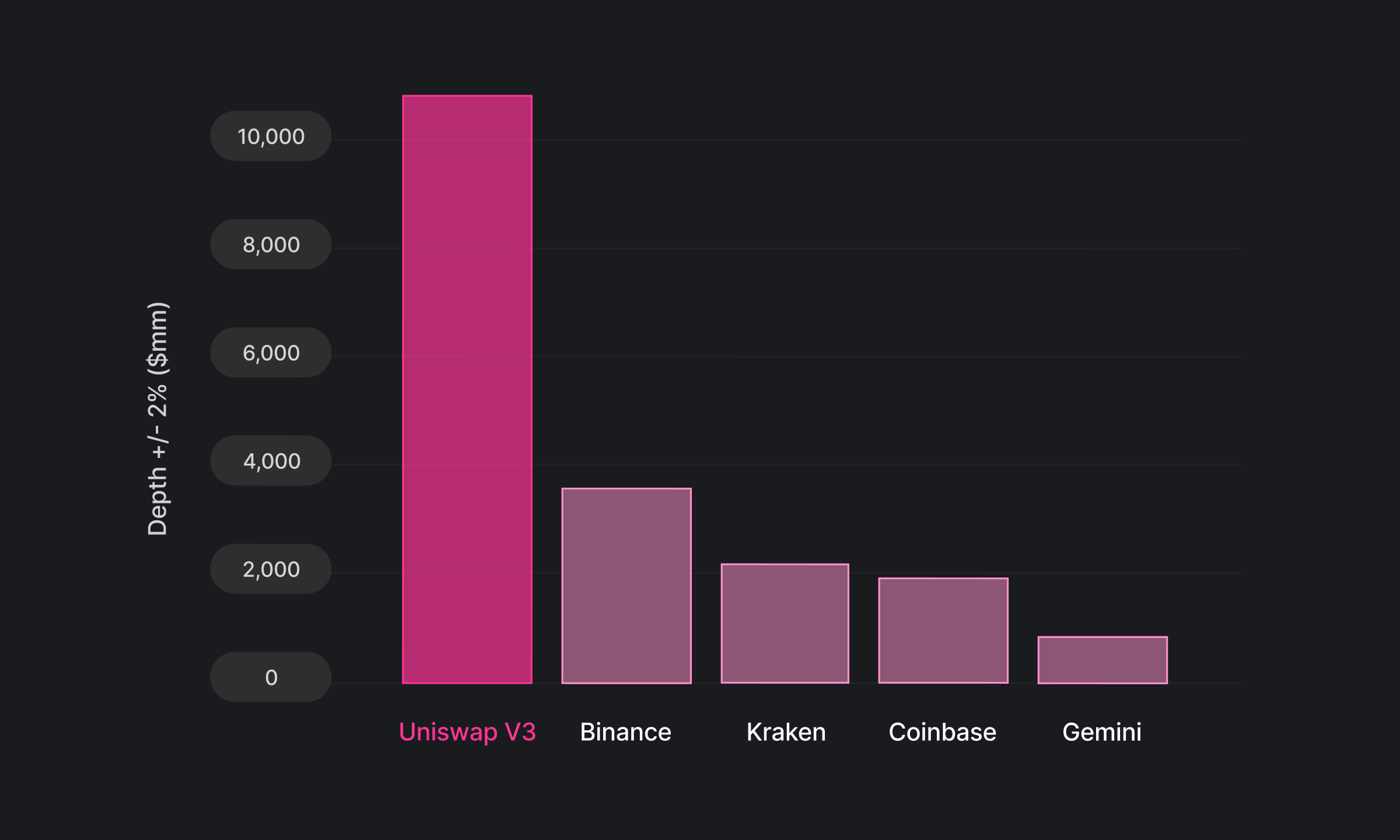This figure compares the market depth for the ETH/(W)BTC on Uniswap v3, Binance, and Kraken and Gemini. Data on centralized exchanges is provided by Kaiko. Sample period is from 02/28/22 to 03/28/22.

## Appendix B - Methodology

We describe the methodology for calculating market depth in Uniswap V2 (constant product AMM) and Uniswap V3 below.

Let $m(\delta)$ be market depth defined as the amount of asset $x$ that can be exchanged for asset $y$ for a given $\delta$ percent of price impact (e.g. $\delta=2%)$. Let $p_0$ be the current spot price of asset $x$ in units of asset $y$, and $L$ be the liquidity amount locked in the pool. Market depth for Uniswap V2 expressed in units of asset $x$ is calculated as

$m(\delta)=\left|\frac{L}{\sqrt{\left(1+\delta\right)p_{0}}}-\frac{L}{\sqrt{_0}}\right|.$

Market depth calculation in Uniswap V3 is more complicated as the liquidity distribution is an aggregation of individual liquidity provider positions. Let $\lambda_{x}(i)$ be the amount of asset $x$ locked in the liquidity range between tick $i$ and $i+s$, where $s$ is the tick spacing for the pool. Let $\lambda_{y}(i)$ be the amount of asset $y$ in this tick range. It can be shown that for lower tick $i$ and associated lower bound prices $p_a$ and upper bound price $p_b$, assets $x$ and $y$ locked in the tick spacing are

$\lambda_{x}(i)=\frac{L}{\sqrt{z}}-\frac{L}{\sqrt{p_{b}}}$

and

$\lambda_{y}(i)=L\left(\sqrt{z}-\sqrt{p_{a}}\right)$ where $z=\begin{cases} p_{a} & \forall p_0\le p_{a}\ p_0 & \forall p_0\in\left(p_{a},p_{b}\right)\ p_{b} & \forall p_0\ge p_{b} \end{cases}.$

Market depth for Uniswap V3 expressed in units of asset $x$ is

$m\left(\delta\right)=\frac{1}{s}\sum_{i=i_{0}}^{i_{0}+d(\delta)}\left|\lambda_x(i)+p_0^{-1}\lambda_y(i)\right|,$ where $i_0$ is the current tick associated with $p_0$ and $d$ is the tick-equivalent of the percentage price change $\delta$.

Derivation for V2 market depth

Let $m(\delta)$ be market depth defined as the amount of asset $x$ that can be exchanged for asset $y$ for a given $\delta$ percent of price impact (e.g. $\delta=2%$). The bonding curve is described by $xy=L^{2}$.

Using the price relationship $p =\frac{y}{x}$, we can also express the bonding curve as $p =\frac{L^{2}}{x^{2}}$,$p =\frac{y^{2}}{L^{2}}$,$x =\frac{L}{\sqrt{p}}$, or $y=L\sqrt{p}$.

After a trade of $\Delta x$ units of asset $x$ for $\Delta y$ units of asset $y$, the bonding curve is

$\left(x+\Delta x\right)(y+\Delta y)=L^{2}.$ We solve for $\Delta x$ s.t. $p_{1}/p_{0}-1=\delta$, substituting $p_0=y/x$ and $p_1=(y+\Delta y)/(x+\Delta x)$, the expression becomes $\frac{y+\Delta y}{x+\Delta x}\frac{x}{y}-1=\delta.$

Substitute into the post-trade equation, we get $\left(x+\Delta x\right)^{2}\left(\delta+1\right)p_{0}=L^{2}.$

Market depth in units of asset $x$ is therefore $m(\delta)\equiv |\Delta x|=\left|\frac{L}{\sqrt{\left(1+\delta\right)p_{0}}}-\frac{L}{\sqrt{p_0}}\right|$

Derivation for V3 market depth

Let $j$ be an index for lower tick boundary and $s$ be the tick spacing (e.g. $s=60$). Let $p_0$ be the current price and $p_{a}$, $p_{b}$ be the prices associated with upper and lower ticks of a tick-space range. More explicity, $p_a=1.0001^j$ and $p_b=1.0001^{(j+s)}$.

Starting with real reserve curve of a single position, Equation 2.2 from v3 white paper:

$\left(x+\frac{L}{\sqrt{p_{b}}}\right)\left(y+L\sqrt{p_{a}}\right)=L^{2}$ For $p_0\le p_{a}$, $x =\frac{L}{\sqrt{p_{a}}}-\frac{L}{\sqrt{p_{b}}}=L\frac{\sqrt{p_{b}}-\sqrt{p_{a}}}{\sqrt{p_{a}p_{b}}}$ $y =0$ For $p_0\ge p_{b}$, $x =0$ $y =L\left(\sqrt{p_{b}}-\sqrt{p_{a}}\right)$ For $p_0\in\left(p_{a},p_{b}\right)$, $x =\frac{L}{\sqrt{p_0}}-\frac{L}{\sqrt{pb}}=L\frac{\sqrt{p_{b}}-\sqrt{p_0}}{\sqrt{p_0p_{b}}}$ $y =L\left(\sqrt{P}-\sqrt{p_{a}}\right)$

This can be written more concisely as

$x =\frac{L}{\sqrt{z}}-\frac{L}{\sqrt{p_{b}}}=\frac{\sqrt{p_{b}}-\sqrt{z}}{\sqrt{sp_{b}}}$ $y =L\left(\sqrt{z}-\sqrt{p_{a}}\right)$ where $z=\begin{cases} p_{a} & \forall P\le p_{a}\ p_0 & \forall P\in\left(p_{a},p_{b}\right)\ p_{b} & \forall P\ge p_{b} \end{cases}$

Let i be the integer index identifying a tick. Let $\delta$ be percentage change in price and $d$ be the associated change in ticks such that $\delta =\frac{p_{1}}{p_{0}}-1=\frac{1.0001^{i_{0}+d}}{1.0001^{i_{0}}}-1$

Let $\lambda_{x}(i)$ be the amount of asset $x$ locked in the liquidity range between tick $i$ and $i+s$, where $s$ is the tick spacing for the pool. Let $\lambda_{y}(i)$ be the amount of asset $y$ in this tick range. It can be shown that for lower tick $i$ and associated lower bound prices $p_a$ and upper bound price $p_b$, assets $x$ and $y$ locked in the tick spacing are $\lambda_{x}(i)=\frac{L}{\sqrt{z}}-\frac{L}{\sqrt{p_{b}}}$

and

$\lambda_{y}(i)=L\left(\sqrt{z}-\sqrt{p_{a}}\right),$ with $z$ defined above.

Market depth for Uniswap V3 expressed in units of asset $x$ is

$m\left(\delta\right)=\frac{1}{s}\sum_{i=i_{0}}^{i_{0}+d(\delta)}\left|\lambda_x(i)+p_0^{-1}\lambda_y(i)\right|,$ where $i_0$ is the current tick associated with $p_0$ and $d$ is the tick-equivalent of the percentage price change $\delta$.